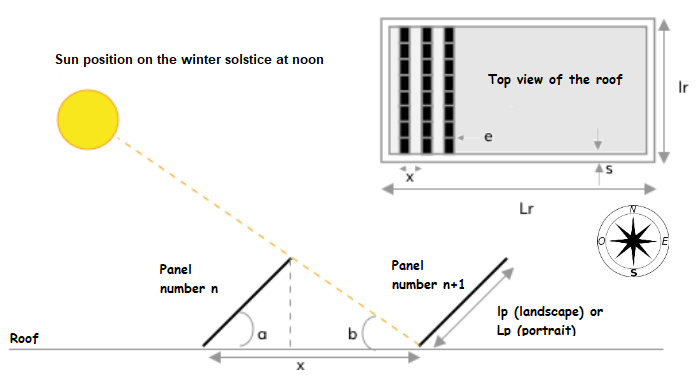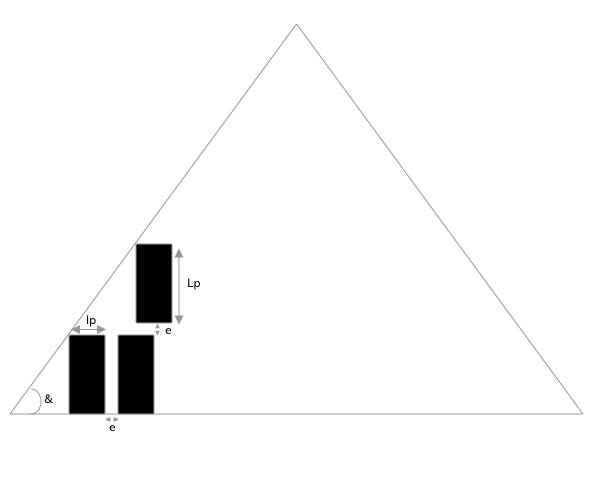# How does MyDualsun determine the maximum number of installable panels on a roof?

In order to determine the maximum number of panels that can be installed on your roof, our MyDualSun simulation software is based on the length and width of the selected panel because each panel has specific dimensions that you can find in their technical sheet, available here : Technical documentation.

We must also distinguish the type of roof : flat roof, rectangle roof or triangle roof.

The surface of the panel is then compared to the surface of your roof, taking into account the spacing required between the panels. In the case of a roof terrace, we calculate the spacing between each panel according to the degree of inclination of your roof so that there is no shadow on the panels in the worst angle of inclination of the sun. : the winter solstice. Then we apply a safe distance to the edges of your roof.

We compare the maximum number of panels that can be installed in portrait and landscape and we keep the higher number of panels to offer it to you.

We will use in the following descriptions the following variables:

• Lr : roof length
• lr : roof width
• Lp : length of the DualSun panel
• lp : DualSun panel width
• a : inclination of the flat roof panels
• b : angle of the sun during the winter solstice at noon
• e : spacing between the frames of 2 successive panels. The value used by default is 2 cm.
• x : spacing between 2 rows of flat roof panels
• s : buffer margin between the edge of the roof and the 1st panel on the flat roof. The value depends on the roof, the parapet and the certifications of the installation systems. You can consult our ATEC SOPRASOLAR FIX EVO certification. We will take here the value of 1 meter recommended by Soprasolar.
• Nmax : maximum number of installable panels
• B2 : short length in the case of a trapezoidal roof

## On a flat roofThe algorithm is as follows.

To calculate the spacing between 2 rows of flat roof panels, you can use the following formulas :

• x = (1+tan(a)tan(b)).cos(a).lp  in landscape
• x = (1+tan(a)tan(b)).cos(a).Lp  in portrait

Then you can calculate the number of panels that can be installed on your roof by rounding down to the lower whole number of the formula below :

• Nmax = Lr - 2.sLp + e . lr-2.sx

## In a rectangular pitched roof

You can calculate the number of panels that can be installed on your roof by rounding down to the lower whole number of the formula below :

• Nmax = Lrlp + e . lrLp + e in portrait
• Nmax = LrLp + e . lrlp + e in landscape

## In a trapezoidal or triangle pitched roof

The calculation is quite similar for the 2 types of roof. We must think of the trapezium as being included in a triangle.tan(&) = 2.lrLr.tof   for an isosceles triangle roof

tan(&) = 2.lrLr-B2  for a trapezoidal roof

The algorithm is as follows:

• We try to fit a panel of width lp on the roof at the bottom left, taking into account a safety margin
• As long as a panel fits, we add one to its right
• As soon as we can no longer fit in, we go to the top line
• And we start again